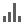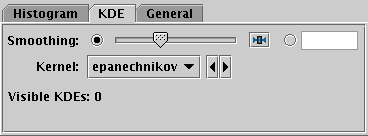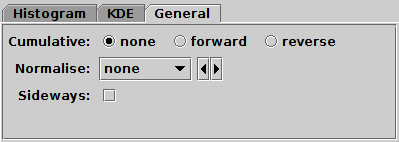Next Previous Up Contents
Next: Plane Plot Window
Up: Histogram Plot Window
Previous: Histogram Axes Control

#### A.4.7.3 Bins Control

The Bins control () is found in the control stack of the Histogram window. It configures the common placement and calculation of some options for all the histogram-like layers displayed. Being able to set these values in common for all displayed layers of a similar type can be convenient, but if you need to use different parameters for different datasets, you can plot the same layer forms in the Plane window (which has no fixed Bins control) instead.

There are three tabs: Histogram, KDE and General.Histogram tab of histogram window Bins fixed control

The Histogram tab affects all Histogram layers, and to some extent the Gaussian layer, and has the following controls:

Bin Size
A scale for the width of bins that are shown on the screen. There are two ways to specify this. If the left-hand radio button is selected, the adjacent slider will adjust the bin size, which is also affected by the actual width of the plotting window in pixels. Slide the slider left to get narrower bins or right to get wider ones. If the right-hand radio button is selected, you can enter a numeric value giving the actual width in data units of each bar (for a logarithmic X axis this value is a factor).

Although Gaussian layers don't have bars, the value of this control can affect the scaling of plotted gaussian fits for some normalisation options, since the Gaussian plots try to scale themselves to match the height of corresponding histograms.

Bin Phase
Controls where the horizontal zero point for binning is set. For instance if your bin size is 1, it controls whether bin boundaries are at 0, 1, 2, .. or 0.5, 1.5, 2.5, ... etc. If the slider is at either end of the scale, there will be a bin boundary at X=0 (linear X axis) or X=1 (logarithmic X axis).KDE tab of histogram window Bins fixed control

The KDE (Kernel Density Estimate) tab affects all KDE, KNN and Densogram layers, and has the following controls:

Smoothing
Configures the smoothing width for kernel density estimation. This is the characteristic width of the kernel function to be convolved with the density to produce the visible plot.

Sliding the slider to the right makes the kernel width larger. The width in data units is shown in the text field on the right (if the X axis is logarithmic, this is a factor). Alternatively you can click the radio button near the text field, and enter the width in data units directly.

Note this affects KDE and Densogram layers, but not KNN layers, which have their own smoothing controls.

Kernel
The functional form of the smoothing kernel. The functions listed refer to the unscaled shape; all kernels are normalised to give a total area of unity.

The available options are:

• square: Uniform value: f(x)=1, |x|=0..1
• linear: Triangle: f(x)=1-|x|, |x|=0..1
• epanechnikov: Parabola: f(x)=1-x*x, |x|=0..1
• cos: Cosine: f(x)=cos(x*pi/2), |x|=0..1
• cos2: Cosine squared: f(x)=cos^2(x*pi/2), |x|=0..1
• gauss3: Gaussian truncated at 3.0 sigma: f(x)=exp(-x*x/2), |x|=0..3
• gauss6: Gaussian truncated at 6.0 sigma: f(x)=exp(-x*x/2), |x|=0..6General tab of histogram window Bins fixed control

The General tab affects all histogram-like layers, and has the following controls:

Cumulative
If set to forward or reverse, the bin values are calculated cumulatively; each bin includes the counts from all previous bins in the direction of negative or positive infinity.
Normalise
Defines how, if at all, the bars are normalised. The available options are:
• none: No normalisation is performed.
• area: The total area of histogram bars is normalised to unity. For cumulative plots, this behaves like height.
• unit: Histogram bars are scaled by the inverse of the bin width in data units. For cumulative plots, this behaves like none.
• maximum: The height of the tallest histogram bar is normalised to unity. For cumulative plots, this behaves like height.
• height: The total height of histogram bars is normalised to unity.

Next Previous Up Contents
Next: Plane Plot Window
Up: Histogram Plot Window
Previous: Histogram Axes Control

TOPCAT - Tool for OPerations on Catalogues And Tables